Collaborations/Challenges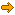Pixel Joint Forum : Pixel Art : Collaborations/ChallengesTopic: CHALLENGE 6/23/2014: Rubik's Vision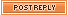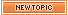Oldest Post First Newest Post First << Prev Page  1 2  of 2
 Author Message jalonso Admiral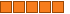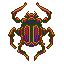Joined: 12 August 2020 Online Status: Offline Posts: 13537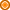Posted: 28 June 2014 at 9:30am First thing first.AA is used to smooth that which pure pixels cannot do.Ideally you want to just pixel purely and use technique variations as a last resort.What you did in your update is cool because you used AA to correct/smooth what is 'technically' a bad line.What I was trying to get at is that there are other options to be pure and use no technique such as  AA if you plan the of use visual tricks to work for you.In my edit the line angles are not correct or line up at all but the grid fools the eye. I used the foreground grid or bars or whatever they are to hide every jaggy and the isolated bits you can't then hide by force. Of course the angle of the light rays will change which in this case it did not seem to matter much so its a smarter design choice. Sometimes you can't and have to AA. Its all about the pixel at hand.Edited by jalonso - 28 June 2014 at 9:32am PJs FAQ <> Sticky Reads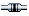IP Logged AirStyle Commander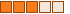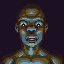Joined: 13 November 2017 Online Status: Offline Posts: 376Posted: 28 June 2014 at 11:48am I see what you mean, sensei, and I saw what that was doing to my lines. I'm glad that I wasn't actually too far off what you were saying. I did try that, and I found that messed with the trajectory of the light rays too much for me. So, I went with AA. Turning all the lines into a 1:1 line with it breaking behind the bars made it change course slightly, and I wanted the light ray to appear to be expanding over time.Doing that messed with their appearance too much. However, I do like what it did the light frame (or whatever the technical phrase is; the light window). It worked with that very well.I see what you've done, and I've already applied my personal fix to the project. I will remember what you showed me, and apply it to future pieces.The last thing I want to cover before I stop monopolizing this forum is the finishing touches: thisI was struggling with this a lot more than I thought I would, so I wanted some feedback. I used this as a reference.see< style="height: 80px; width: 444px; border: 1px solid grey; padding: 2px;"><>< value="af">Afrikaans< value="sq">Albanian< value="ar">Arabic< value="hy">Armenian< value="az">Azerbaijani< value="eu">Basque< value="be">Belarusian< value="bg">Bulgarian< value="ca">Catalan< value="zh-CN">Chinese (Simplified)< value="zh-TW">Chinese (Traditional)< value="hr">Croatian< value="cs">Czech< value="da">Danish< value="auto" ed="ed">Detect language< value="nl">Dutch< value="en">English< value="et">Estonian< value="tl">Filipino< value="fi">Finnish< value="fr">French< value="gl">Galician< value="ka">Georgian< value="de">German< value="el">Greek< value="ht">Haitian Creole< value="iw">Hebrew< value="hi">Hindi< value="hu">Hungarian< value="is">Icelandic< value="id">Indonesian< value="ga">Irish< value="it">Italian< value="ja">Japanese< value="ko">Korean< value="la">Latin< value="lv">Latvian< value="lt">Lithuanian< value="mk">Macedonian< value="ms">Malay< value="mt">Maltese< value="no">Norwegian< value="fa">Persian< value="pl">Polish< value="pt">Portuguese< value="ro">Romanian< value="ru">Russian< value="sr">Serbian< value="sk">Slovak< value="sl">Slovenian< value="es">Spanish< value="sw">Swahili< value="sv">Swedish< value="th">Thai< value="tr">Turkish< value="uk">Ukrainian< value="ur">Urdu< value="vi">Vietnamese< value="cy">Welsh< value="yi">Yiddish⇄<>< value="af">Afrikaans< value="sq">Albanian< value="ar">Arabic< value="hy">Armenian< value="az">Azerbaijani< value="eu">Basque< value="be">Belarusian< value="bg">Bulgarian< value="ca">Catalan< value="zh-CN">Chinese (Simplified)< value="zh-TW">Chinese (Traditional)< value="hr">Croatian< value="cs">Czech< value="da">Danish< value="nl">Dutch< value="en" ed="ed">English< value="et">Estonian< value="tl">Filipino< value="fi">Finnish< value="fr">French< value="gl">Galician< value="ka">Georgian< value="de">German< value="el">Greek< value="ht">Haitian Creole< value="iw">Hebrew< value="hi">Hindi< value="hu">Hungarian< value="is">Icelandic< value="id">Indonesian< value="ga">Irish< value="it">Italian< value="ja">Japanese< value="ko">Korean< value="la">Latin< value="lv">Latvian< value="lt">Lithuanian< value="mk">Macedonian< value="ms">Malay< value="mt">Maltese< value="no">Norwegian< value="fa">Persian< value="pl">Polish< value="pt">Portuguese< value="ro">Romanian< value="ru">Russian< value="sr">Serbian< value="sk">Slovak< value="sl">Slovenian< value="es">Spanish< value="sw">Swahili< value="sv">Swedish< value="th">Thai< value="tr">Turkish< value="uk">Ukrainian< value="ur">Urdu< value="vi">Vietnamese< value="cy">Welsh< value="yi">YiddishEnglish (auto-detected) » EnglishIP Logged jalonso AdmiralJoined: 12 August 2020 Online Status: Offline Posts: 13537Posted: 28 June 2014 at 12:04pm There is monopolizing this forum, eva! What is that for, the preview? PJs FAQ <> Sticky ReadsIP Logged AirStyle CommanderJoined: 13 November 2017 Online Status: Offline Posts: 376Posted: 28 June 2014 at 12:21pm Yes, the small detail photo. As you may have well surmised, this project is...how should I say...freaking big. So I made a small one, so that it would actually show up on the front page for a while, without me having to change my avatar.UPDATE:I decided to do this instead:< style="height: 80px; width: 444px; border: 1px solid grey; padding: 2px;"><>< value="af">Afrikaans< value="sq">Albanian< value="ar">Arabic< value="hy">Armenian< value="az">Azerbaijani< value="eu">Basque< value="be">Belarusian< value="bg">Bulgarian< value="ca">Catalan< value="zh-CN">Chinese (Simplified)< value="zh-TW">Chinese (Traditional)< value="hr">Croatian< value="cs">Czech< value="da">Danish< value="auto" ed="ed">Detect language< value="nl">Dutch< value="en">English< value="et">Estonian< value="tl">Filipino< value="fi">Finnish< value="fr">French< value="gl">Galician< value="ka">Georgian< value="de">German< value="el">Greek< value="ht">Haitian Creole< value="iw">Hebrew< value="hi">Hindi< value="hu">Hungarian< value="is">Icelandic< value="id">Indonesian< value="ga">Irish< value="it">Italian< value="ja">Japanese< value="ko">Korean< value="la">Latin< value="lv">Latvian< value="lt">Lithuanian< value="mk">Macedonian< value="ms">Malay< value="mt">Maltese< value="no">Norwegian< value="fa">Persian< value="pl">Polish< value="pt">Portuguese< value="ro">Romanian< value="ru">Russian< value="sr">Serbian< value="sk">Slovak< value="sl">Slovenian< value="es">Spanish< value="sw">Swahili< value="sv">Swedish< value="th">Thai< value="tr">Turkish< value="uk">Ukrainian< value="ur">Urdu< value="vi">Vietnamese< value="cy">Welsh< value="yi">Yiddish⇄<>< value="af">Afrikaans< value="sq">Albanian< value="ar">Arabic< value="hy">Armenian< value="az">Azerbaijani< value="eu">Basque< value="be">Belarusian< value="bg">Bulgarian< value="ca">Catalan< value="zh-CN">Chinese (Simplified)< value="zh-TW">Chinese (Traditional)< value="hr">Croatian< value="cs">Czech< value="da">Danish< value="nl">Dutch< value="en" ed="ed">English< value="et">Estonian< value="tl">Filipino< value="fi">Finnish< value="fr">French< value="gl">Galician< value="ka">Georgian< value="de">German< value="el">Greek< value="ht">Haitian Creole< value="iw">Hebrew< value="hi">Hindi< value="hu">Hungarian< value="is">Icelandic< value="id">Indonesian< value="ga">Irish< value="it">Italian< value="ja">Japanese< value="ko">Korean< value="la">Latin< value="lv">Latvian< value="lt">Lithuanian< value="mk">Macedonian< value="ms">Malay< value="mt">Maltese< value="no">Norwegian< value="fa">Persian< value="pl">Polish< value="pt">Portuguese< value="ro">Romanian< value="ru">Russian< value="sr">Serbian< value="sk">Slovak< value="sl">Slovenian< value="es">Spanish< value="sw">Swahili< value="sv">Swedish< value="th">Thai< value="tr">Turkish< value="uk">Ukrainian< value="ur">Urdu< value="vi">Vietnamese< value="cy">Welsh< value="yi">YiddishEnglish (auto-detected) » English < style="height: 80px; width: 444px; border: 1px solid grey; padding: 2px;"><>< value="af">Afrikaans< value="sq">Albanian< value="ar">Arabic< value="hy">Armenian< value="az">Azerbaijani< value="eu">Basque< value="be">Belarusian< value="bg">Bulgarian< value="ca">Catalan< value="zh-CN">Chinese (Simplified)< value="zh-TW">Chinese (Traditional)< value="hr">Croatian< value="cs">Czech< value="da">Danish< value="auto" ed="ed">Detect language< value="nl">Dutch< value="en">English< value="et">Estonian< value="tl">Filipino< value="fi">Finnish< value="fr">French< value="gl">Galician< value="ka">Georgian< value="de">German< value="el">Greek< value="ht">Haitian Creole< value="iw">Hebrew< value="hi">Hindi< value="hu">Hungarian< value="is">Icelandic< value="id">Indonesian< value="ga">Irish< value="it">Italian< value="ja">Japanese< value="ko">Korean< value="la">Latin< value="lv">Latvian< value="lt">Lithuanian< value="mk">Macedonian< value="ms">Malay< value="mt">Maltese< value="no">Norwegian< value="fa">Persian< value="pl">Polish< value="pt">Portuguese< value="ro">Romanian< value="ru">Russian< value="sr">Serbian< value="sk">Slovak< value="sl">Slovenian< value="es">Spanish< value="sw">Swahili< value="sv">Swedish< value="th">Thai< value="tr">Turkish< value="uk">Ukrainian< value="ur">Urdu< value="vi">Vietnamese< value="cy">Welsh< value="yi">Yiddish⇄<>< value="af">Afrikaans< value="sq">Albanian< value="ar">Arabic< value="hy">Armenian< value="az">Azerbaijani< value="eu">Basque< value="be">Belarusian< value="bg">Bulgarian< value="ca">Catalan< value="zh-CN">Chinese (Simplified)< value="zh-TW">Chinese (Traditional)< value="hr">Croatian< value="cs">Czech< value="da">Danish< value="nl">Dutch< value="en" ed="ed">English< value="et">Estonian< value="tl">Filipino< value="fi">Finnish< value="fr">French< value="gl">Galician< value="ka">Georgian< value="de">German< value="el">Greek< value="ht">Haitian Creole< value="iw">Hebrew< value="hi">Hindi< value="hu">Hungarian< value="is">Icelandic< value="id">Indonesian< value="ga">Irish< value="it">Italian< value="ja">Japanese< value="ko">Korean< value="la">Latin< value="lv">Latvian< value="lt">Lithuanian< value="mk">Macedonian< value="ms">Malay< value="mt">Maltese< value="no">Norwegian< value="fa">Persian< value="pl">Polish< value="pt">Portuguese< value="ro">Romanian< value="ru">Russian< value="sr">Serbian< value="sk">Slovak< value="sl">Slovenian< value="es">Spanish< value="sw">Swahili< value="sv">Swedish< value="th">Thai< value="tr">Turkish< value="uk">Ukrainian< value="ur">Urdu< value="vi">Vietnamese< value="cy">Welsh< value="yi">YiddishEnglish (auto-detected) » EnglishEdited by AirStyle - 28 June 2014 at 1:38pmIP Logged frank62899 Seaman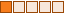Joined: 29 June 2014 Online Status: Offline Posts: 1Posted: 29 June 2014 at 8:02am First time posting so i don't know how to submit this to the challenge... Anyways any directions on how to submit it would be appreciated! On to the art its supposed to be the viper from Battlestar Galactica and i think it turned out okay. Only used 8 colors.IP Logged Red-Error Seaman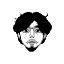Joined: 02 May 2010 Online Status: Offline Posts: 15Posted: 29 June 2014 at 12:34pm Originally posted by frank62899First time posting so i don't know how to submit this to the challenge... Anyways any directions on how to submit it would be appreciated! On to the art its supposed to be the viper from Battlestar Galactica and i think it turned out okay. Only used 8 colors.You should log-in and submit it here http://www.pixeljoint.com Upper-left : Gallery-> Submit Pixel-art and Check the "Submit to weekly challenge" box http://www.pixeljoint.comEdited by Red-Error - 29 June 2014 at 12:35pmIP Logged jalonso AdmiralJoined: 12 August 2020 Online Status: Offline Posts: 13537Posted: 29 June 2014 at 1:17pm @frank62899 (assuming 6/28/99 is your bday...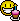)Please take time to read and review the stickied posts in the resource section of the forum and the FAQ thread.To answer your question. Read this for explanations. Notice the weekly challenge check box.PJs FAQ <> Sticky ReadsIP Logged
 << Prev Page  1 2  of 2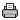Printable version

Forum Jump
You cannot post new topics in this forum
You cannot reply to topics in this forum
You cannot delete your posts in this forum
You cannot edit your posts in this forum
You cannot create polls in this forum
You cannot vote in polls in this forum